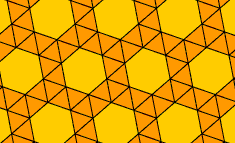A# Archimedean tessellationAn Archimedean tessellation, also known as a semiregular tessellation, is a tiling that uses only regular polygons arranged so that two or more different polygons are around each vertex and each vertex involves the same pattern of polygons. There are eight such tessellations, two involving triangles and squares, two involving triangles and hexagons, and one each involving squares and octagons; triangles and dodecagons; squares, hexagons and dodecagons; and triangles, squares and hexagons.